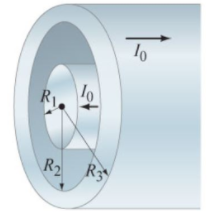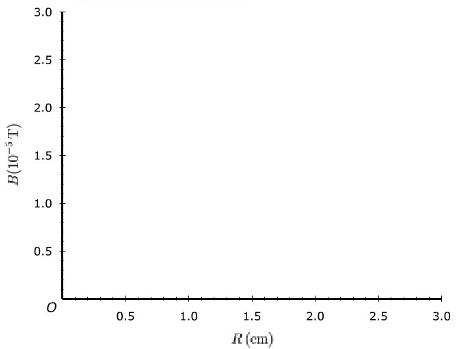# Problem: A coaxial cable consists of a solid inner conductor of radius R1 surrounded by a concentric cylindrical tube of inner radius R2 and outer radius R3 (the figure). The conductors carry equal and opposite currents Io distributed uniformly across their cross sections (Figure 1).Let Io = 1.50 A, R1 = 1.00 cm, R2 = 2.00 cm, and R3 = 2.50 cm. Graph B from R = 0 to R = 3.00 cm.

###### FREE Expert Solution

In this problem, we are dealing with the magnetic field produced by straight currents. We'll use amperes law to determine the magnetic field around a current.

Ampere's law:

$\overline{){\mathbf{\oint }}{\mathbf{B}}{\mathbf{·}}{\mathbf{dl}}{\mathbf{=}}{{\mathbf{\mu }}}_{{\mathbf{0}}}{{\mathbf{I}}}_{\mathbf{e}\mathbf{n}\mathbf{c}\mathbf{l}\mathbf{o}\mathbf{s}\mathbf{e}\mathbf{d}}}$

B•∫dl = μ0Ienc

91% (34 ratings)###### Problem Details

A coaxial cable consists of a solid inner conductor of radius R1 surrounded by a concentric cylindrical tube of inner radius R2 and outer radius R3 (the figure). The conductors carry equal and opposite currents Io distributed uniformly across their cross sections (Figure 1).Let Io = 1.50 A, R1 = 1.00 cm, R2 = 2.00 cm, and R3 = 2.50 cm. Graph B from R = 0 to R = 3.00 cm.Frequently Asked Questions

What scientific concept do you need to know in order to solve this problem?

Our tutors have indicated that to solve this problem you will need to apply the Magnetic Field Produced by Straight Currents concept. You can view video lessons to learn Magnetic Field Produced by Straight Currents. Or if you need more Magnetic Field Produced by Straight Currents practice, you can also practice Magnetic Field Produced by Straight Currents practice problems.

What professor is this problem relevant for?

Based on our data, we think this problem is relevant for Professor Shaevitz's class at Barnard College.